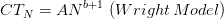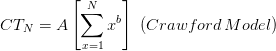# Learning Curve Calculator

This learning curve calculator provides a quick and straightforward method of calculating the total cost or time it will take for a certain repetitive process in a manufacturing environment at a distinguished learning rate, the time at which the first unit will be produced, and the number of units.

Learning Curve Calculator

## Understanding the Learning Curve

In the manufacturing context, the experience curve or learning curve refers to the way in which the efficiency of processes increases over time as the units are produced. The learning rate or learning curve coefficient refers to the amount of money that is saved every time production is doubled.

For instance, let's say the time it takes to produce a given number of units falls by 20% for every double in production numbers; as such, the learning curve percentage is 80%.

Two models can be employed to determine how much time it will take to manufacture N units at a given learning rate and the time required to produce the first unit: the Wright Model and the Crawford Model. The equations associated with each model are similar. If A represents the time taken to manufacture the first unit, the learning curve factor is b ( b = ln(learning percentage / 100) / ln(2) ), then the cumulative total time (CTN) required to manufacture N units is as follows:NASA provided the following guidelines in relation to the learning curve:

• If the process involves 75% hand labor and 25% machine labor, the learning percent is in the region of 80%.
• If the process requires equal hand labor and machine labor (50% each), the learning percent is in the region of 85%.
• If the process involves 75% machine labor and 25% hand labor, the learning percent is in the region of 90%.

The closer the learning percent moves toward 100%, the less significant the effects of experience. Where there is a high percentage of manual labor in a process, the lower the learning curve percent.